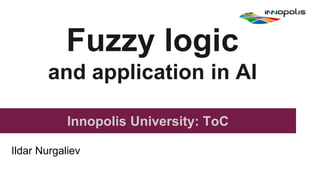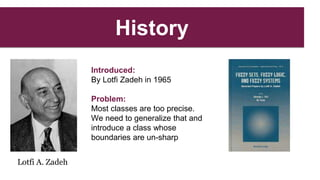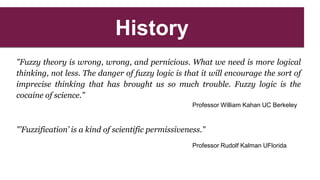Ce diaporama a bien été signalé.
Le téléchargement de votre SlideShare est en cours. ×

# Fuzzy logic and application in AI

Publicité
Publicité
Publicité
Publicité
Publicité
Publicité
Publicité
Publicité
Publicité
Publicité
PublicitéChargement dans…3
×

## Consultez-les par la suite

1 sur 46 Publicité

# Fuzzy logic and application in AI

Fuzzy logic: from start to application.
- hystory
- first order logic
- second order logic
-linguistique variable
- applicaion in IT

Fuzzy logic: from start to application.
- hystory
- first order logic
- second order logic
-linguistique variable
- applicaion in IT

Publicité
Publicité

### Fuzzy logic and application in AI

1. 1. Fuzzy logic and application in AI Innopolis University: ToC Ildar Nurgaliev
2. 2. History Lotfi A. Zadeh Introduced: By Lotfi Zadeh in 1965 Problem: Most classes are too precise. We need to generalize that and introduce a class whose boundaries are un-sharp
3. 3. History "Fuzzy theory is wrong, wrong, and pernicious. What we need is more logical thinking, not less. The danger of fuzzy logic is that it will encourage the sort of imprecise thinking that has brought us so much trouble. Fuzzy logic is the cocaine of science." Professor William Kahan UC Berkeley "’Fuzzification’ is a kind of scientific permissiveness." Professor Rudolf Kalman UFlorida
4. 4. History
5. 5. Classical logic Proposition - a sentence with a truth value. Truth value - true (1 or T) or false (0 or F)
6. 6. Classical logic Logic operations:
7. 7. Classical logic Logic operations:
8. 8. Classical logic Logic formula: ● Each propositional variable is a formula ● If X is a formula, then ¬X is a formula ● If X and Y are formulas, then X * Y is a formula, where * is any binary connective
9. 9. Fuzzy logic Truth value ranges between 0 (completely false) and 1 ( completely true).
10. 10. Fuzzy logic Logic operations: ¬X = (1-truth(X)) X AND Y = minimum(truth(X), truth(Y)) X OR Y = maximum(truth(X), truth(Y)) X ⊃ Y = maximum(minimum(truth(X),truth(Y)),1-truth(X))
11. 11. First order logic -Fuzzy modifier -Fuzzy Truth quantifier -Linguistic variable -Fuzzy predicate
12. 12. Predicate A “predicate” is a group of words like applies to Objects Socrates Tree Two That hat Predicates is a man is green is less than belongs to
13. 13. Fuzzy Predicate A fuzzy predicate is a predicate whose definition contains ambiguity “z is expensive.” “w is young.”
14. 14. How to interpret Fuzzy Predicate? -P(x) is a fuzzy set. -evaluated by membership function μP(x) “x is P” x - variable P - ambiguity set
15. 15. Membership function Example: Watson used these functions for reasoning degree of true Conjunction rule: μA⋀B(x)=min[μA(x), μB(x)] Disjunction rule: μA⋁B(x)=max[μA(x), μB(x)] Negation rule: μㄱA(x)=1-μA(x)
16. 16. Fuzzy Modifier “w is young.”
17. 17. Fuzzy Modifier “w is young.” add the modifier “very”
18. 18. Fuzzy Modifier “w is young.” add the modifier “very”
19. 19. Fuzzy Modifier T(“Age”) = {young, very young, very very young, … } μvery young(u) = (μyoung(u))2 “w is young.” add the modifier “very”
20. 20. Linguistic variable Linguistic variable = (x, T(x), U, G, M)
21. 21. Linguistic variable Linguistic variable = (x, T(x), U, G, M) x: name of variable
22. 22. Linguistic variable Linguistic variable = (x, T(x), U, G, M) x: name of variable T(x): set of linguistic terms which can be a value of the variable
23. 23. Linguistic variable Linguistic variable = (x, T(x), U, G, M) x: name of variable T(x): set of linguistic terms which can be a value of the variable U: set of universe of discourse which defines the characteristics of the variable
24. 24. Linguistic variable Linguistic variable = (x, T(x), U, G, M) x: name of variable T(x): set of linguistic terms which can be a value of the variable U: set of universe of discourse which defines the characteristics of the variable G: syntactic grammar which produces terms in T(x)
25. 25. Linguistic variable Linguistic variable = (x, T(x), U, G, M) x: name of variable T(x): set of linguistic terms which can be a value of the variable U: set of universe of discourse which defines the characteristics of the variable G: syntactic grammar which produces terms in T(x) M: semantic rules which map terms in T(x) to fuzzy sets in U
26. 26. Linguistic variable
27. 27. Linguistic variable X={“Hot”, T(Hot), U,G(Hot), M(hot)} name : “Hot”, T(Hot) : (‘warm’, ‘hot’, ‘very hot’), U : ( [0..100] ), G(Hot) : { ‘warm’ } ∪ { ‘hot’ } ∪ Ti+1 = { ‘very’ . Ti } ) M(hot) = { (u, μhot(u)) | u •∊ U } ps: Hot - linguistic variable hot - predicate
28. 28. Linguistic variable Directions: 1. Empty contents into saucepan; add 4½ cups (1 L) cold water. 2. Bring to a boil, stirring constantly. 3. Reduce heat; partially cover and simmer for 15 minutes, stirring occasionally. 4 to 6 servings, 4½ cups (1 L) Example: Linguistic variables in soup instructions
29. 29. Fuzzy Truth Values The qualifiers in T define “fuzzy truth values” and they can be defined by the μP(x) (membership functions).
30. 30. Fuzzy Truth Values fuzzy truth qualifier is defined in the universal set U = {Q | Q ∊ [0,1]}. The qualifiers in T define “fuzzy truth values” and they can be defined by the μP(x) (membership functions).
31. 31. Fuzzy Truth Values fuzzy truth qualifier is defined in the universal set U = {Q | Q ∊ [0,1]}. T = {true, very true, fairly true, absolutely true, … , absolutely false, fairly false, false} The qualifiers in T define “fuzzy truth values” and they can be defined by the μP(x) (membership functions).
32. 32. Truth qualifiers by μP(x)
33. 33. LETS START PARTY! The Application of Fuzzy logic in AI -Knowledge Base (KB)
34. 34. Inference and Knowledge Representation rule type: if-then
35. 35. Inference and Knowledge Representation (1) Modus ponens Fact: x is A Rule: If (x is A) then (y is B) Result: y is B rule type: if-then
36. 36. Inference and Knowledge Representation (1) Modus ponens Fact: x is A Rule: If (x is A) then (y is B) Result: y is B (2) Modus tollens Fact: y is ㄱB Rule: If (x is A) then (y is B) Result: x is ㄱA ps: The modus ponens is used in the forward inference and the modus tollens is in the backward one. rule type: if-then
37. 37. Representation of Fuzzy Predicate “x is P”, it is represented by: - fuzzy set P(x) - membership function μP(x)(x)
38. 38. Representation of Fuzzy Predicate “x is P”, it is represented by: - fuzzy set P(x) - membership function μP(x)(x) Fuzzy Relation R = { ( x, μR(x)) | μR(x) ⩾ 0, x •∊ A}
39. 39. Representation of Fuzzy Predicate “x is P”, it is represented by: - fuzzy set P(x) - membership function μP(x)(x) Fuzzy Relation R = { ( x, μR(x)) | μR(x) ⩾ 0, x •∊ A} Represent a predicate by fuzzy relation: R(x) = P
40. 40. Representation of Fuzzy Rule If x is A, then y is B or If A(x), then B(y)
41. 41. Representation of Fuzzy Rule If x is A, then y is B or If A(x), then B(y) R(x, y): If A(x), then B(y) or R(x, y): A(x)➝B(y) R = { ( (x, y), μR(x, y)) | μR(x, y) ⩾ 0, x •∊ A, y ∊ B}
42. 42. Inference (1) Generalized modus ponens (GMP) Fact: x is A : R(x) Rule: If (x is A) then (y is B) : R(x, y) Result: y is B : R(y) = R(x) o R(x, y)
43. 43. Inference Example KB: (x is A)➝(y is B), x is A I R(x,y) = A × B II fact ‘x is A’ into the form R(x) III R(y) = R(x) o R(x, y) μR(y) = ⋁ [μR(x) š⋀ μR(x, y)] x
44. 44. Inference Example KB: (x is A)➝(y is B), x is A
45. 45. Fuzzy logic application ● household appliances ● animation systems ● industrial automation ● chemical industry ● aerospace ● robotics ● mining and metal processing ● transportation
46. 46. Thank you for attention References: 1) ‘First Course on Fuzzy Theory and Applications’, Kwang H. Lee 2) ‘Linguistic Variables: Clear Thinking with Fuzzy Logic’, Walter Banks

### Notes de l'éditeur

• … Classical logic
proposition: a sentence having truth value true (1) or false (0)
truth value: proposition o {0, 1}
logic variable: variable representing a proposition
… Logic operation
negation (NOT):CP
conjunction (AND): a š b
disjunction (OR): a › b
implication (o): a o b
… Logic function
logic function
logic primitive
logic formula

Fuzzy logic
fuzzy logic formula
fuzzy proposition 214 8. Fuzzy Logic
truth value: fuzzy proposition o [0, 1]
… Fuzzy logic operation
negation (NOT):CP
conjunction (AND): a š b
disjunction (OR): a › b
implication (o): Min(1, 1 b a)
• Tautology
tautology: logic formula whose value is always true
inference: developing new facts by using the tautology
… Deductive inference
modus ponens
modus tollens
hypothetical syllogism
… Predicate logic
predicate
predicate logic
predicate proposition: proposition consist of predicate and object
evaluation of proposition
… Quantifier
universal quantifier: (for all)
existential quantifier: (there exists)

… Fuzzy predicate
fuzzy predicate: predicate represented by fuzzy sets
fuzzy truth value [0, 1]
fuzzy modifier
… Fuzzy truth qualifier
fuzzy truth value: true, very true, fairly true, etc.
Pvery true(v) = (true(v))2
value of “P is very true” is 0.81 when value of P is 0.9
• As we know now, a predicate proposition in the classical logic has the
following form.
“x is a man.”
“y is P.”
x and y are variables, and “man” and “P” are crisp sets. The sets of
individuals satisfying the predicates are written by “man(x)” and “P(y)”.
Definition (Fuzzy predicate) .A fuzzy predicate is a predicate whose
definition contains ambiguity Ƒ
Example 8.16 For example,
“z is expensive.”
“w is young.”
The terms “expensive” and “young” are fuzzy terms. Therefore the
sets “expensive(z)” and “young(w)” are fuzzy sets. Ƒ
When a fuzzy predicate “x is P” is given, we can interpret it in two
ways.
(1) P(x) is a fuzzy set. The membership degree of x in the set P is
defined by the membership function PP(x).
(2) PP(x) is the satisfactory degree of x for the property P. Therefore, the
truth value of the fuzzy predicate is defined by the membership
function.
Truth value = PP(x)
• The membership function of a fuzzy set is a generalization of the indicator function in classical sets. In fuzzy logic, it represents the degree of truth as an extension of valuation. Degrees of truth are often confused with probabilities, although they are conceptually distinct, because fuzzy truth represents membership in vaguely defined sets, not likelihood of some event or condition.

ps: In mathematics, an indicator function or a characteristic function is a function defined on a set X that indicates membership of an element in a subset A of X, having the value 1 for all elements of A and the value 0 for all elements of X not in A.
• Knowledge Base is a physical system so we have to represent Predicate to another form for presenting in KB.
For this reason we use Fuzzy Relation.
• The fuzzy rule may include fuzzy predicates in the antecedent and
consequent,
• The operation used in the
reasoning is denoted by the notation “o”, and thus the result is represented
by the output of the composition when we use the GMP.

• Fuzzy Relation